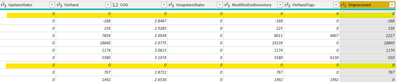cancel
Showing results for
Did you mean:New Member

## Filter rows if multiple columns have values are 0

How do I filter out rows that have zeros in multiple columns?1 ACCEPTED SOLUTIONSuper User

You can create a custom column with rule of

if Coulmn1 = 0 and

Column2 = 0 and

Column3 = 0 and

etc.

then "Hide"

else "Show"

then filter the custom column to Show.

Hope this helps

3 REPLIES 3Super User

Put following formula in a custom column which will give TRUE if all columns in a rows are 0. Then you can choose FALSE to filter out TRUE rows.

``= List.MatchesAll(Record.ToList(_),each _=0)``

See the working here - Open a blank query - Home - Advanced Editor - Remove everything from there and paste the below code to test

``````let
Source = Table.FromRows(Json.Document(Binary.Decompress(Binary.FromText("i45WMlTSUYIgYygrVidayQDIwMQgGSDDBK7FFKw+FgA=", BinaryEncoding.Base64), Compression.Deflate)), let _t = ((type nullable text) meta [Serialized.Text = true]) in type table [Column1 = _t, Column2 = _t, Column3 = _t, Column4 = _t, Column5 = _t, Column6 = _t]),
#"Changed Type" = Table.TransformColumnTypes(Source,{{"Column1", Int64.Type}, {"Column2", Int64.Type}, {"Column3", Int64.Type}, {"Column4", Int64.Type}, {"Column5", Int64.Type}, {"Column6", Int64.Type}}),
#"Filtered Rows" = Table.SelectRows(#"Added Custom", each ([Custom] = false)),
#"Removed Columns" = Table.RemoveColumns(#"Filtered Rows",{"Custom"})
in
#"Removed Columns"``````Super User

You can create a custom column with rule of

if Coulmn1 = 0 and

Column2 = 0 and

Column3 = 0 and

etc.

then "Hide"

else "Show"

then filter the custom column to Show.

Hope this helpsNew Member

I ended up using the following filter on one of the number columns:

if Coulmn1 <> 0 or

Column2 <> 0 or

Column3 <> 0 or

etc.